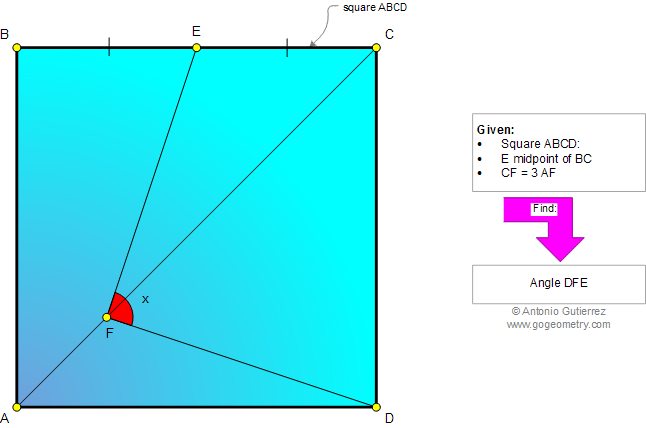# Geometry Problem 932: Square, Midpoint, Diagonal, Ratio 3:1, Angle Measure, Congruence.

< PREVIOUS PROBLEM  |  NEXT PROBLEM >

 In a square ABCD, E is the midpoint of BC and F is a point on AC so that CF = 3AF. Find the measure of angle DFE.